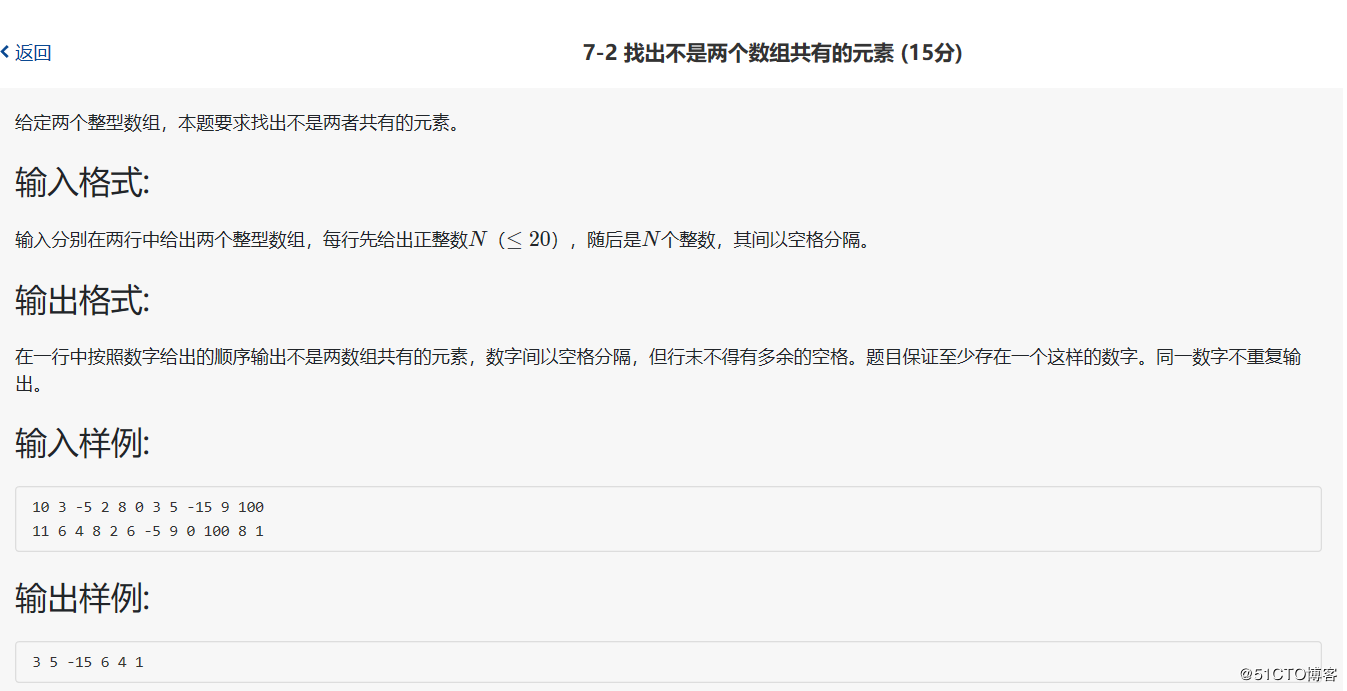• 2022-01-23 22:39:18

给定两个整型数组，本题要求找出不是两者共有的元素。

### 输入格式:

输入分别在两行中给出两个整型数组，每行先给出正整数N（≤20），随后是N个整数，其间以空格分隔。

### 输出格式:

在一行中按照数字给出的顺序输出不是两数组共有的元素，数字间以空格分隔，但行末不得有多余的空格。题目保证至少存在一个这样的数字。同一数字不重复输出。

### 输入样例:

10 3 -5 2 8 0 3 5 -15 9 100
11 6 4 8 2 6 -5 9 0 100 8 1


### 输出样例:

3 5 -15 6 4 1

直接看代码！！！

#include<stdio.h>
int main()
{
int m,n,a,b,c={0},d={0};
int i,cnt,j,t,z,p,flag,k;
scanf("%d",&m);
for(i=0;i<m;i++)
scanf("%d",&a[i]);

scanf("%d",&n);
for(i=0;i<n;i++)
scanf("%d",&b[i]);
cnt=0;
for(i=0;i<m;i++)
for(j=0;j<n;j++)
if(a[i]==b[j])
{
c[cnt]=a[i];cnt++;
}
t=0;
for(i=0;i<m;i++)
{
flag=0;
for(j=0;j<cnt;j++)
if(a[i]==c[j])
{
flag=1;break;
}
if(flag==0)
{d[t]=a[i];t++;}
}
z=t;
for(i=0;i<n;i++)
{
flag=0;
for(j=0;j<cnt;j++)
if(b[i]==c[j])
{
flag=1;break;
}
if(flag==0)
{
d[z]=b[i];z++;
}
}
for(i=0;i<z;i++)
{
flag=0;
for(j=0;j<i;j++)//注意我们要先输出数组前面的元素，所以要用该元素后的元素跟他比来判断是否重复
{
if(d[i]==d[j])
{
flag=1;break;
}
}
if(flag==0&&i!=(z-1))printf("%d ",d[i]);
if(flag==0&&i==(z-1))printf("%d",d[i]);
}
return 0;
}

这题思路不难，就是写起来有点费手指头，我连想带写花了整整一个小时，唉，还是太菜了 。

其实这题的代码可以再简化一下，将第一个数组和第二个数组相比找出不是共有的元素，然后再用第二个数组和第一个数组比较找出不是共有的元素，然后就可以得出来数组D了奥~c语言
更多相关内容
• #includeint main(){int m;while (scanf("%d", &m) !=EOF){int a, i;for (i = 0; i < m; i++)scanf("%d", &a[i]);int n;scanf("%d", &n);int b;for (i = 0; i < n;......#include

int main()

{

int m;

while (scanf("%d", &m) !=EOF)

{

int a, i;

for (i = 0; i < m; i++)

scanf("%d", &a[i]);

int n;

scanf("%d", &n);

int b;

for (i = 0; i < n; i++)

scanf("%d", &b[i]);

int j;

int c, k;

k = 0;

for (i = 0; i < m; i++)

{

for (j = 0; j < n; j++)

{

if (a[i] == b[j])

break;

}

if (j >= n)

{

c[k] = a[i];

k++;

}

}

for (i = 0; i < n; i++)

{

for (j = 0; j < m; j++)

{

if (b[i] == a[j])

break;

}

if (j >= m)

{

c[k] = b[i];

k++;

}

}

printf("%d", c);

for (i = 1; i < k; i++)

{

for (j = 0; j < i; j++)

{

if (c[i] == c[j])

break;

}

if (j >= i)

printf(" %d", c[i]);

}

printf("\n");

}

return 0;

}

展开全文• 练习7-4 找出不是两个数组共有的元素 (20 分) 给定两个整型数组，本题要求找出不是两者共有的元素。 输入格式: 输入分别在两行中给出两个整型数组，每行先给出正整数N（≤20），随后是N个整数，其间以空格分隔。 ...

## 题目

练习7-4 找出不是两个数组共有的元素 (20 分)
给定两个整型数组，本题要求找出不是两者共有的元素。

输入格式:
输入分别在两行中给出两个整型数组，每行先给出正整数N（≤20），随后是N个整数，其间以空格分隔。

输出格式:
在一行中按照数字给出的顺序输出不是两数组共有的元素，数字间以空格分隔，但行末不得有多余的空格。题目保证至少存在一个这样的数字。同一数字不重复输出。

输入样例:
10 3 -5 2 8 0 3 5 -15 9 100
11 6 4 8 2 6 -5 9 0 100 8 1
输出样例:
3 5 -15 6 4 1

## 分析

有两个条件，1.按照数字给出的顺序输出不是两数组共有的元素，2.同一数字不重复输出。

不改变原始顺序的去重：删去一个重复元素后，修改整个大循环的限定条件。

通常来说for循环的条件表达式是一个固定的数，但这里它根据需要进行了调整。

## 代码

#include<stdio.h>

int main(){
int n,m,i,j,a,b,c,k=0;
scanf("%d",&n);
for(i=0;i<n;++i){
scanf("%d",&a[i]);
}
scanf("%d",&m);
for(i=0;i<m;++i){
scanf("%d",&b[i]);
}

for(i=0;i<n;++i){//查第一个数组有，第二个数组无的元素
for(j=0;j<m;++j){
if(a[i]==b[j]) break;
}
if(j==m){
c[k]=a[i];
k++;
}
}

for(i=0;i<m;++i){//查第二个数组有，第一个数组无的元素
for(j=0;j<n;++j){
if(a[j]==b[i]) break;
}
if(j==n){
c[k]=b[i];
k++;
}
}
int l;
for(i=0;i<k;++i){//数组去重，不改变原始顺序，并更新数组
for(j=i+1;j<k;++j){
if(c[i]==c[j]){
for(l=j+1;l<k;++l){
c[l-1]=c[l];
}
k=k-1;//重复的去掉，因此数组长度减一
}
}
}
for(i=0;i<k;++i){
if(i==0)
printf("%d",c[i]);
else
printf(" %d",c[i]);
}
}

展开全文• 题目：给定两个整型数组，本题要求找出不是两者共有的元素。 源码： #include <stdio.h> int main(void) { int m,n,i,j,k,flag1 = 1,cnt = 0,flag2 = 1,b = {0}; //数组b用于存放要输出的元素 scanf("%d...

题目：给定两个整型数组，本题要求找出不是两者共有的元素。源码：

#include <stdio.h>
int main(void)
{
int m,n,i,j,k,flag1 = 1,cnt = 0,flag2 = 1,b = {0};     //数组b用于存放要输出的元素

scanf("%d",&m);

int a1[m];

for(i = 0;i < m;i++)
scanf("%d",&a1[i]);          //输入第一个数组

scanf("%d",&n);

int a2[n];

for(i = 0;i < n;i++)
scanf("%d",&a2[i]);          //输入第二个数组

for(i = 0;i < m;i++)
{
flag1 = 1;
for(j = 0;j < n;j++)
{
if(a1[i] == a2[j])
{
flag1 = 0;
break;
}
}							//逐个找出a1中有而a2中没有的元素
if(flag1)
{
for(k = 0;k < cnt;k++)
{
flag2 = 1;
if(a1[i] == b[k])
{
flag2 = 0;
break;
}
}
if(flag2)
{
cnt++;
b[cnt - 1] = a1[i];
}						//将a1中有而a2中没有的元素与要输出的元素进行比较，没有相同的则留下
}
}

for(j = 0;j < n;j++)
{
flag1 = 1;
for(i = 0;i < m;i++)
{
if(a2[j] == a1[i])
{
flag1 = 0;
break;
}
}							//逐个找出a2中有而a1中没有的元素
if(flag1)
{
for(k = 0;k < cnt;k++)
{
flag2 = 1;
if(a2[j] == b[k])
{
flag2 = 0;
break;
}
}
if(flag2)
{
cnt++;
b[cnt - 1] = a2[j];
}						//将a1中有而a2中没有的元素与要输出的元素进行比较，没有相同的则留下
}
}

for(i = 0;i < cnt;i++)
if(i)
printf(" %d",b[i]);
else
printf("%d",b[i]);

return 0;
}展开全文c语言
• 输入分别在两行中给出两个整型数组，每行先给出正整数N（≤20），随后是N个整数，其间以空格分隔。 输出格式: 在一行中按照数字给出的顺序输出不是数组共有的元素，数字间以空格分隔，但行末不得有多余的空格。...散列表
• 输入分别在两行中给出两个整型数组，每行先给出正整数N（≤20），随后是N个整数，其间以空格分隔。 输出格式: 在一行中按照数字给出的顺序输出不是数组共有的元素，数字间以空格分隔，但行末不得有多余的空格。...c语言
• 实验7-1-8 找出不是两个数组共有的元素 题目描述 给定两个整型数组，本题要求找出不是两者共有的元素。 输入 输入分别在两行中给出两个整型数组，每行先给出正整数N（≤20），随后是N个整数，其间以空格分隔。 ...c语言
• 输入分别在两行中给出两个整型数组，每行先给出正整数N（≤20），随后是N个整数，其间以空格分隔。 输出格式: 在一行中按照数字给出的顺序输出不是数组共有的元素，数字间以空格分隔，但行末不得有多余的空格。...c语言
• 输入分别在两行中给出两个整型数组，每行先给出正整数N（≤20），随后是N个整数，其间以空格分隔。 输出格式: 在一行中按照数字给出的顺序输出不是数组共有的元素，数字间以空格分隔，但行末不得有多余的空格。...
• 该题大概思路容易想到，找不是两个数组共有的元素倒是挺好，但是具体实现起来也是不大容易，多个输入时调试也挺麻烦的，主要做起来有个比较麻烦的地方。就是不是两个数组共有的元素有两个，但是最终只能够输出一...c语言
• 输入分别在两行中给出两个整型数组，每行先给出正整数N（≤20），随后是N个整数，其间以空格分隔。 输出格式: 在一行中按照数字给出的顺序输出不是数组共有的元素，数字间以空格分隔，但行末不得有多余的空格。...java
• 输入分别在两行中给出两个整型数组，每行先给出正整数N（≤20），随后是N个整数，其间以空格分隔。 输出格式: 在一行中按照数字给出的顺序输出不是数组共有的元素，数字间以空格分隔，但行末不得有多余的空格。...
• 7-9 找出不是两个数组共有的元素 (20 分) C++c++
• 输入分别在两行中给出两个整型数组，每行先给出正整数N（≤20），随后是N个整数，其间以空格分隔。 输出格式: 在一行中按照数字给出的顺序输出不是数组共有的元素，数字间以空格分隔，但行末不得有多余的空格。...c语言
• 找出不是两个数组共有的元素 给定两个整型数组，本题要求找出不是两者共有的元素。 输入格式: 输入分别在两行中给出两个整型数组，每行先给出正整数N（≤20），随后是N个整数，其间以空格分隔。 输出格式: 在一行中...
• 练习7-8 找出不是两个数组共有的元素（20 分）c语言
• 给定两个整型数组，本题要求找出不是两者共有的元素。c语言
• 7-5 找出不是两个数组共有的元素 (20分) 给定两个整型数组，本题要求找出不是两者共有的元素。 输入格式: 输入分别在两行中给出两个整型数组，每行先给出正整数N（≤20），随后是N个整数，其间以空格分隔。 输出格式...c语言 算法 数据结构
• 输入分别在两行中给出两个整型数组，每行先给出正整数N（≤20），随后是N个整数，其间以空格分隔。 输出格式: 在一行中按照数字给出的顺序输出不是数组共有的元素，数字间以空格分隔，但行末不得有多余的空格。...c语言
• 本文首发于微信公众号："算法与编程之美"，欢迎关注，及时了解更多此系列文章。前言数在日常生活中，我们常常需要去辨别两个事物的不同之处，现在我们可以尝试用程序去找出两组数据...算法 python java 编程语言 数据结构
• 输入分别在两行中给出两个整型数组，每行先给出正整数N（≤20），随后是N个整数，其间以空格分隔。 输出格式: 在一行中按照数字给出的顺序输出不是数组共有的元素，数字间以空格分隔，但行末不得有多余的空格。...
• <!DOCTYPE html> <html lang="en"> <head> <meta charset="UTF-8"> <title>Document</title> </head> <body> <script type="text/... var b=[6 ,4, 8, 2,
• 输入分别在两行中给出两个整型数组，每行先给出正整数N（≤20），随后是N个整数，其间以空格分隔。 输出格式: 在一行中按照数字给出的顺序输出不是数组共有的元素，数字间以空格分隔，但行末不得有多余的空格。...c语言 开发语言 后端
• 输入分别在两行中给出两个整型数组，每行先给出正整数N（≤20），随后是N个整数，其间以空格分隔。 输出格式: 在一行中按照数字给出的顺序输出不是数组共有的元素，数字间以空格分隔，但行末不得有多余的空格。...python list
• 输入分别在两行中给出两个整型数组，每行先给出正整数N（≤20），随后是N个整数，其间以空格分隔。 输出格式: 在一行中按照数字给出的顺序输出不是数组共有的元素，数字间以空格分隔，但行末不得有多余的空格。...c语言 开发语言 后端
• 找出不是两个数组共有的元素 如： input 10 3 -5 2 8 0 3 5 -15 9 100 11 6 4 8 2 6 -5 9 0 100 8 1 output 3 5 -15 6 4 1 #include <stdio.h> #include <stdlib.h> int main() { int m; while(~......